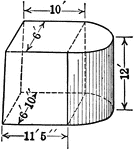### Composite Figure of Quadrilateral Frustum With Half of a Cylinder Attached

An illustration of a composite figure made up of a quadrilateral frustum and half of a cylinder. Frustum…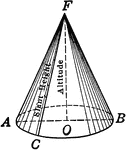### Circular Cone

An illustration of a circular cone.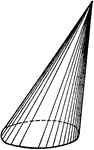### Right Circular Cone

An illustration of a right circular cone with labels on slant height and altitude.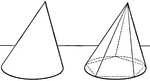### Volume of Cone

Illustration of a cone with a polygon inscribed used to show that the volume of a circular cone is equal…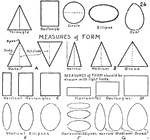### Measures of Form

Labeled shapes showing various measures of form.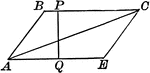### Altitude and Diagonal of a Parallelogram

Illustration of a parallelogram with altitude and diagonal drawn.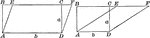### Area of a Parallelogram

Illustration of parallelogram AEFC drawn two different ways with base b and altitude/height a used to…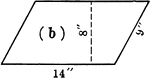### Parallelogram With Dimensions

Parallelogram with dimensions labeled. Parallelogram can be used to calculate area.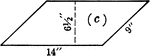### Parallelogram With Dimensions

Parallelogram with dimensions labeled. Parallelogram can be used to calculate area.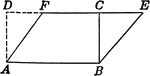### Parallelogram and Rectangle Relationship

Parallelogram with dimensions drawn to show relationship to the area of a rectangle.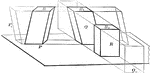### Volume of Parallelopiped

Diagram used to prove the theorem: "The volume of a any parallelopiped is equal to the product of its…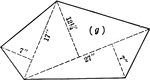### Pentagon With Triangular Sections For Area

Pentagon with dimensions labeled. Pentagon can be used to calculate area by calculating individual triangles…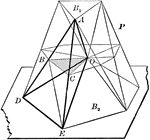### Volume of Prismatoid

Diagram used to prove the theorem: "The volume of a prismatoid is equal to the product of one-sixth…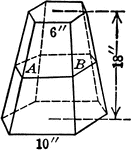### Pyramid Frustum With Hexagonal Bases and 6 inch and 10 inch Sides

An illustration of a pyramid with the top cut off by a plane parallel to the base. The remaining part…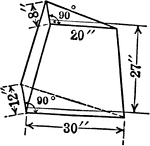### Pyramid Frustum With Triangular Bases and Height of 27 inches

An illustration of a pyramid with the top cut off by a plane parallel to the base. The remaining part…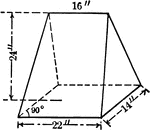### Pyramid Frustum With Triangular Bases and Height of 27 inches

An illustration of a pyramid with the top cut off by a plane parallel to the base. The remaining part…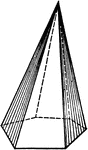### Pyramid With Hexagonal Base

An illustration of a pyramid with a hexagonal base.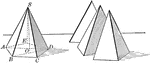### Pentagonal Pyramid For Volume

Illustration of pentagonal pyramid used to show that the volume is equal to one third of the product…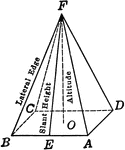### Regular Pyramid With Square Base

An illustration of a regular pyramid with a square base and labels on lateral edge, slant height, altitude,…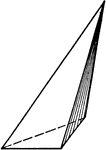### Pyramid With Triangular Base

An illustration of a pyramid with a triangular base.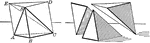### Triangular Pyramid For Volume

Illustration of triangular pyramid used to show that the volume is equal to one third of the product…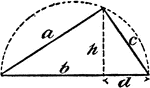### Right Triangle Inscribed In A Semicircle

An illustration of a right triangle inscribed in a semicircle.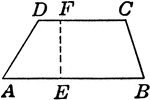### Trapezoid

Trapezoid with angles and height labeled.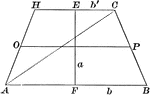### Area of a Trapezoid

Illustration of a trapezoid with altitude a and bases b and b' used to demonstrate that the area is…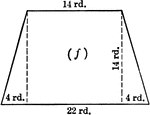### Trapezoid With Dimensions

Trapezoid with dimensions labeled. Trapezoid can be used to calculate area.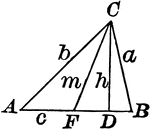### Triangle With Height and Median

Triangle with height/altitude h and median m.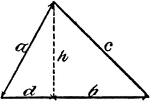### Acute Triangle

An illustration of an acute triangle with the height/altitude labeled h.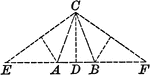### Triangle With Altitude and Isosceles Triangles Within

Illustration showing a triangle with an altitude CD passing through the middle of EF. Triangles AEC…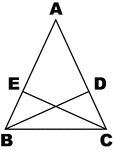### Altitudes Drawn from Base Angles of Isosceles Triangles

Illustration to show altitudes drawn from base angles of an isosceles triangle.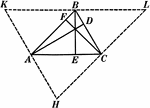### Altitudes In A Triangle

Illustration used to show "The altitudes of a triangle are concurrent."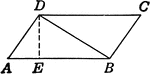### Area of Triangle

Parallelogram illustration to show where the area of a triangle formula comes from.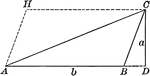### Area of a Triangle

Illustration of triangle ABC with altitude a and base b to be used to demonstrate that the area is 1/2ba.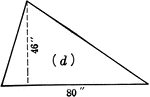### Triangle With Dimensions

Triangle with dimensions labeled. Triangle can be used to calculate area.### Triangle With Dimensions

Triangle with dimensions labeled. Triangle can be used to calculate area.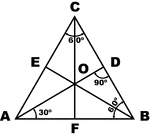### Equilateral Triangle

Sketch of equilateral triangle with bisectors, medians, and altitudes.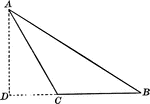### Obtuse Triangle

Obtuse triangle with altitude drawn.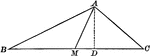### Obtuse Triangle

Obtuse triangle with altitude and one median drawn.### Obtuse Triangle With Height Drawn

Triangle with sides a,b,c and height/altitude h.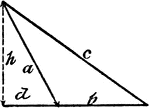### Obtuse Triangle

An illustration of an obtuse triangle with the height/altitude labeled h.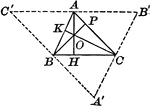### Orthocenter of Triangle

Illustration to show altitudes in a triangle. It is known as the orthocenter.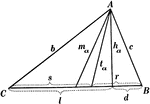### Parts Of A Triangle

Illustration used to show the various parts of a triangle: sides, angles, medians, altitudes, bisectors,…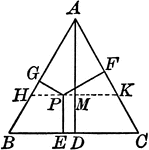### Perpendiculars Dropped Any Point in the Equilateral Triangle

Illustration to show the perpendiculars dropped from any point in the equilateral triangle to the three…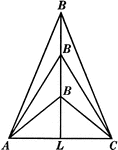### 3 Isosceles Triangles Wit Equal Bases

Illustration of three isosceles triangles with the same base AC but varying heights (as B gets farther…### Similar Triangles With Altitudes Drawn

Illustration that shows two similar triangles with altitudes drawn.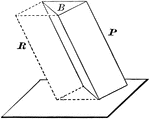### Volume of Triangular Prism

Diagram used to prove the theorem: "The volume of a triangular prism is equal to the product of its…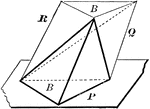### Volume of Triangular Pyramid

Diagram used to prove the theorem: "The volume of a triangular pyramid is equal to one third of a triangular…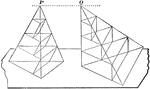### Equivalent Triangular Pyramids

Diagram used to prove the theorem: "Two triangular pyramids having equivalent bases and equal altitudes…